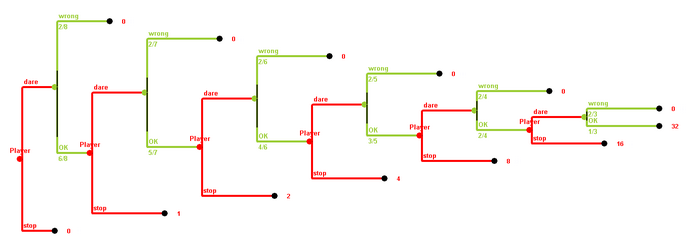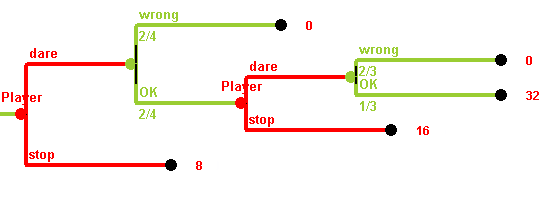# Probability: Decision Theory

The tree we consider is no longer a probability tree, with situations and different experiments at the situations. Rather only in some situations randome experiments are performed. At other situations the human player is supposed to make decisions, to choose between several options. Therefore we have two types of vertices in the tree or digraph---those where "Nature moves", where experiments are performed, and those where the human moves. For those where the human moves, edges are drawn towards the possible resulting situations.

Quiz Show: You play a quiz show with up to 6 questions. The questions are worth \$1, \$1, \$2, \$4, \$8, and \$16. After each round, you have the option to stop and take the money you have won so far, or hear the next question. If you have heared the next question, there is no way out---you have to answer it correctly, to go into the next round. If you give the wrong answer, all your money is lost. You also know that in round n the probability of giving a wrong answer is 2/(9-n). (Thus it increases from 2/8 over 2/7, 2/6, 2/5, 2/4 to 2/3 over the six rounds.) When do you stop?

Here is the tree. At each round you have the option to dare the next question or to stop. If you stop, an edge goes to a leaf, an end situation, and red number written at that leaf shows how much you have won. If you dare the next question, an edge goes to a green vertex, where a random experiment is performed. This random experiment has two outcomes---the answer can be correct or wrong. The probabilities for the two oucomes are written below the green edge. If the answer was wrong, we have again reached a leaf, a final situation, with payoff of 0. Otherwise, you are facing the decision whether to stop or to dare the next question of the next round.What we want to do is to assign expected payoffs to all vertices of the tree, not just to the leaves. However, if we want to do this by beginning at the beginning we face some problems. What is the expected payoff for the game at the very beginning? Probably it is best to "dare" the first question. Then the payoff for the first decision vertex is the same as the expected payoff at the first green (random) vertex. But this expected payoff would be 2/8·0 + 6/8· the expected payoff of the second player vertex. But this is also unknown, and so on.It makes more sense to try to fill in the missing expected payoffs starting at the end. If you have reached the rightmost green random vertex, i.e. if you have answered the first five questions successfully and dared to answer the last one, your expected payoff will be (2/3)·0 + (1/3)·32 = 32/3. Therefore, at the player's last decision vertex, you have to decide between expected payoff of 32/3 if you dare the last question, and a sure payoff of 16 if you stop. Since 16 > 32/3, it is rational to stop there. Therefore every rational player will stop there, and the expected payoff at that vertex is 16. Now at the second last random vertex, if you are trying to answer the fifth question, your expected payoff is (2/4)·0 + (2/4)·16 = 8. Therefore if you face the decision whether to allow the fifth question (after answering four questions successfully), you face the choice between an expected payoff of 8 if you dare the next qeustion, and a sure payoff of 8 if you stop. We make the assumption here that you always go for the higher payoff, expected or sure, therefore in this case you would be indifferent, but expect 8 at that decision. (Note that in reality things are more complicated. Monetary reward is transfered by so-called utility functions into something which is comparable. Note that almost everybody would prefer sure \$1,000,000 over an expected payoff of the same amount by getting \$2,000,000 with probability 1/2 and nothing with probability 0.)

If you now go one step further to the right, the expected payoff of the next (random) vertex is (2/5)·0 + (3/5)·8 = 24/5, which is higher than the 4 you would get by stopping after the third question. So you would in any case try to answer the fourth question, and never try to answer the last question. If this procedure is continued, we get an expected payoff of 12/7 at the very beginning.

The procedure demonstrated at the example above is called backwards induction. Starting at the leaves to the right, we assign expected payoffs to their predecessors, and the predecessors of that predecessors, and so on, moving from right to left (backwards). Whenever we have a random vertex, we compute the expected value. Whenever we face a decision vertex for the player, we choose the decision leading to the vertex with the highest expected payoff (the maximum), and this maximum is also the expected payoff of our decision vertex in question.

5 Envelopes

\$10000 is distributed into 5 envelopes. One envelope, the "bomb", remains empty. In the basic version the player knows how much is put into each envelope, let's say \$1000, \$2000, \$3000, \$4000, and 0. Then the envelopes are shuffled and in each round you, the player, can either choose to get one of the remaining envelopes or keep the money you have collected so far and quit. If you ask for a new envelope, randomly one of the remaining envelopes is selected and handed to the player. If this envelope contains nothing (the bomb), the player you lose all the money you collected in the previous envelopes, otherwise you add the money to the money taken so far. Here is an Excel analysis of the game.

How much would you "expect" to win when you were playing the game? Would you expect to win more or less if the \$10000 were differently distributed on the envelopes, say as \$2000, \$2000, \$2000, \$2000, and 0? What if it were distributed as \$100, \$500, \$2600, \$7000, and 0? What about other distributions? Would you play different then? And what exactly does "expectation" mean?

Version b: In another version, you, the player can distribute the money into the envelopes (using the requirement that one of them, the bomb, has to remain empty) before starting to play. How would you distribute the money, and how would you play?

Version c: In still another version, the distribution of the money on the 5 envelopes is not known to the player. It is just known that exactly one envelope is empty and that the other four contain all the 10000 Dollar. How would expected value and strategy change? Would they change?

The decision tree of the original 5 Envelopes "game" can be seen here. Since it is so huge, and since the situations where you have drawn first \$2000, and then \$3000, or where you have drawn first \$3000, and then \$2000 are really identical situation for you, it is better to identify such situations and move to the digraph. This digraph can be seen in this Excel sheet,, where backwards induction is done automatically. There are five envelopes, containing amounts A, B, C, D, and 0. You can change the amounts A, B, C, D by typing in different numbers into cells B4 to B7. Actually the random vertices are omitted, all vertices are decision vertices, they are labeled by the bills received so far, and they are colored black if they are final situations (with nothing to decide), red if you rather stop, and green if you rather proceed. The expected value if proceeding is shown in the grey cell below the situation name, and the payoff if stopping, the accumulated payoff, is shown in the yellow cell. In each case, the payoff of that decision vertex is the maximum of both values. Therefore the expected payoff of the original version is \$3200.

Oh-No

You can play ten plays "Oh-No" here.

You are playing in play
You just rolled a
 round 1 round 2 round 3 round 4 round 5 round 6 round 7 round 8 round 9 round 10 Average

The player rolls a die repeatedly. After each roll, as much dollars as the die shows are added to a heap, which initially is empty. The game ends if either

• the player decides that the heap contains enough money, takes the money in the heap, and goes, or
• if a "1" is rolled, in which case all the money already collected in the heap is lost.
Analysis of the "Oh-No" Game: Note that if your sum is n so far, the expected value if you stop is (of course) n, whereas the expected value after the next roll of the die is
0 + (n+2)/6 + (n+3)/5 + (n+4)/6 + (n+5)/6 + (n+6)/6.
When is this value smaller than n? Just for 20 < n. Thus the best strategy is to roll until you have 20 points. Then you stop. The expected value, however, is smaller than 20 if using this optimal strategy. How large is it? What changes in the "6"-version of the game, where a "6" loses? Look at the Excel sheet analysis.
Quiz Show: You play a quiz show with up to 6 questions. The questions are worth \$1, \$2, \$4, \$8, \$16, and \$32. After each round, you have the option to stop and take the money you have won so far, or hear the next question. If you have heared the next question, there is no way out---you have to answer it correctly, to go into the next round. If you give the wrong answer, all your money is lost. You also know that in round n the probability of giving a wrong answer is 2/(9-n). (Thus it increases from 2/8 over 2/7, 2/6, 2/5, 2/4 to 2/3 over the six rounds.) When do you stop?